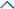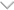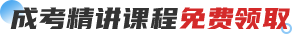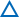欢迎访问长沙成考网！本网站仅为湖南成人高考考生提供湖南成考专升本、高起专、高起本报名咨询以及成考考前辅导服务，官方信息以湖南省教育考试院www.hneeb.cn为准
• 咨询电话：0731-85468015# 2022年长沙成人高考高起本《数学(文)》章节难点习题(1)

2022-05-16 11:41:58 来源： 其它

2022年长沙成人高考考试时间在10月15日和16日进行，请相关考生提前复习备考，长沙成考网为帮助各位考生更好的复习，整理了长沙成人高考高升本模拟试题，如下：

●歼灭难点训练

一、选择题

1.(★★★★)等比数列{an}的首项a1=-1,前n项和为Sn,若 ，则 Sn等于( )

C.2 D.-2

二、填空题

2.(★★★★)已知a,b,a+b成等差数列，a,b,ab成等比数列，且0

3.(★★★★)等差数列{an}共有2n+1项，其中奇数项之和为319，偶数项之和为290，则其中间项为_________.

4.(★★★★)已知a、b、c成等比数列，如果a、x、b和b、y、c都成等差数列，则 =_________.

三、解答题

5.(★★★★★)设等差数列{an}的前n项和为Sn,已知a3=12,S12>0,S13<0.

(1)求公差d的取值范围;

(2)指出S1、S2、…、S12中哪一个值最大，并说明理由.

6.(★★★★★)已知数列{an}为等差数列，公差d≠0,由{an}中的部分项组成的数列

a ,a ,…,a ,…为等比数列，其中b1=1,b2=5,b3=17.

(1)求数列{bn}的通项公式;

(2)记Tn=C b1+C b2+C b3+…+C bn,求 .

7.(★★★★)设{an}为等差数列，{bn}为等比数列，a1=b1=1,a2+a4=b3,b2·b4=a3,分别求出{an}及{bn}的前n项和S10及T10.

8.(★★★★★){an}为等差数列，公差d≠0,an≠0,(n∈N*),且akx2+2ak+1x+ak+2=0(k∈N*)

(1)求证：当k取不同自然数时，此方程有公共根;

(2)若方程不同的根依次为x1,x2,…,xn,…,求证：数列 为等差数列.

参考答案

难点磁场

①

②

解法一：将Sm=30,S2m=100代入Sn=na1+ d,得：

解法二：由 知，要求S3m只需求m[a1+ ],将②-①得ma1+ d=70,∴S3m=210.

解法三：由等差数列{an}的前n项和公式知，Sn是关于n的二次函数，即Sn=An2+Bn(A、B是常数).将Sm=30,S2m=100代入，得

,∴S3m=A·(3m)2+B·3m=210

解法四：S3m=S2m+a2m+1+a2m+2+…+a3m=S2m+(a1+2md)+…+(am+2md)=S2m+(a1+…+am)+m·2md=S2m+Sm+2m2d.

由解法一知d= ,代入得S3m=210.

解法五：根据等差数列性质知：Sm,S2m-Sm,S3m-S2m也成等差数列，从而有：2(S2m-Sm)=Sm+(S3m-S2m)

∴S3m=3(S2m-Sm)=210

解法六：∵Sn=na1+ d,

∴ =a1+ d

∴点(n, )是直线y= +a1上的一串点，由三点(m, ),(2m, ),(3m, )共线，易得S3m=3(S2m-Sm)=210.

解法七：令m=1得S1=30，S2=100，得a1=30,a1+a2=100,∴a1=30,a2=70

∴a3=70+(70-30)=110

∴S3=a1+a2+a3=210

答案：210

歼灭难点训练

一、1.解析：利用等比数列和的性质.依题意， ，而a1=-1,故q≠1,

∴ ,根据等比数列性质知S5，S10-S5，S15-S10,…,也成等比数列，且它的公比为q5,∴q5=- ,即q=- .

∴ 答案：B

二、2.解析：解出a、b,解对数不等式即可.

答案：(-∞,8)

3.解析：利用S奇/S偶= 得解.

答案：第11项a11=29

4.解法一：赋值法.

解法二：

b=aq,c=aq2,x= (a+b)= a(1+q),y= (b+c)= aq(1+q),

= =2.

答案：2

三、5.(1)解：依题意有： 解之得公差d的取值范围为-

(2)解法一：由d<0可知a1>a2>a3>…>a12>a13,因此，在S1，S2，…，S12中Sk为最大值的条件为：ak≥0且ak+1<0,即 ∵a3=12,∴ ，∵d<0,∴2-

因为k是正整数，所以k=6,即在S1，S2，…，S12中，S6最大.

解法二：由d<0得a1>a2>…>a12>a13，因此，若在1≤k≤12中有自然数k,使得ak≥0,且ak+1<0,则Sk是S1，S2，…，S12中的最大值.由等差数列性质得，当m、n、p、q∈N*,且m+n=p+q时，am+an=ap+aq.所以有：2a7=a1+a13= S13<0,∴a7<0,a7+a6=a1+a12= S12>0,∴a6≥-a7>0,故在S1，S2，…，S12中S6最大.

解法三：依题意得： 最小时，Sn最大;

∵-

点评：该题的第(1)问通过建立不等式组求解属基本要求，难度不高，入手容易.第(2)问难度较高，为求{Sn}中的最大值Sk,1≤k≤12,思路之一是知道Sk为最大值的充要条件是ak≥0且ak+1<0，思路之三是可视Sn为n的二次函数，借助配方法可求解.它考查了等价转化的数学思想、逻辑思维能力和计算能力，较好地体现了高考试题注重能力考查的特点.而思路之二则是通过等差数列的性质等和性探寻数列的分布规律，找出“分水岭”，从而得解.

6.解：(1)由题意知a52=a1·a17，即(a1+4d)2=a1(a1+16d) a1d=2d2,

∵d≠0,∴a1=2d,数列{ }的公比q= =3,

∴ =a1·3n-1 ①

又 =a1+(bn-1)d= ②

由①②得a1·3n-1= ·a1.∵a1=2d≠0,∴bn=2·3n-1-1.

(2)Tn=C b1+C b2+…+C bn=C (2·30-1)+C ·(2·31-1)+…+C (2·3n-1-1)= (C +C ·32+…+C ·3n)-(C +C +…+C )= [(1+3)n-1]-(2n-1)= ·4n-2n+ ,

7.解：∵{an}为等差数列，{bn}为等比数列，∴a2+a4=2a3,b2·b4=b32,

已知a2+a4=b3,b2·b4=a3,∴b3=2a3,a3=b32,

得b3=2b32,∵b3≠0,∴b3= ,a3= .

由a1=1,a3= ，知{an}的公差d=- ,

∴S10=10a1+ d=- .

由b1=1,b3= ,知{bn}的公比q= 或q=- ,

8.证明：(1)∵{an}是等差数列，∴2ak+1=ak+ak+2,故方程akx2+2ak+1x+ak+2=0可变为(akx+ak+2)(x+1)=0,

∴当k取不同自然数时，原方程有一个公共根-1.

(2)原方程不同的根为xk=

-281

(一)由于各方面情况的调整与变化本网提供的考试信息仅供参考，敬请以教育考试院及院校官方公布的正式信息为准。

(二）本网注明信息来源为其他媒体的稿件均为转载体，免费转载出于非商业性学习目的，版权归原作者所有。如有内容与版权问题等请与本站联系。联系方式：邮件429504262@qq.com上一篇：2022年长沙成人高考高起本《数学(文)》难点讲解(2)下一篇：2022年长沙成人高考高升本《数学(文)》章节难点习题(2)2022年成考VIP班2021年长沙成人高考考前通关视频！！ 陈老师 36课时 查看详情
2021年长沙成人高考专升本《大学语文》考情分析 雷老师 36课时 查看详情
2021年长沙成人高考专升本《英语》考情分析 吴老师 36课时 查看详情
2021年长沙成人高考专升本《艺术概论》考情分析 36课时 查看详情
2021年长沙成人高考专升本《高等数学》考情分析 36课时 查看详情
2021年长沙成人高考专升本《政治》考情分析 36课时 查看详情
2021年长沙成人高考专升本《教育理论》考情分析 36课时 查看详情2022年成考VIP直播班7天保障畅学无忧

【成考课程学习中心】

• ## 微信公众号## 免费领取说明

1、培训券可兑换一门视频课程。

2、下载注册APP即可获得400培训券，签到、发帖讨论也可获取培训券。

3、鼓励学习，在线学习也可积累培训券。

4、兑换的课程与购买的为相同课程。

5、课程可在APP或者小程序进行学习。

10/21/2023

• ## 微信公众号## 最新文章